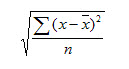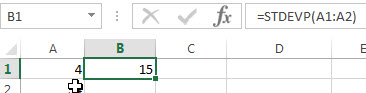# Excel STDEVP Function

This post will guide you how to use the STDEVP function with syntax and examples in Microsoft excel.

### Description

The Excel STDEVP function returns the standard deviation of a population based on an entire population of numbers. This function has been replaced by the STDEV.P function in Excel 2010. The standard deviation is used to identify the amount that a set of values differ from the average value.

The STDEVP function is a build-in function in Microsoft Excel and it is categorized as a Statistical Function.

The STDEVP function is available in Excel 2016, Excel 2013, Excel 2010, Excel 2007, Excel 2003, Excel XP, Excel 2000, Excel 2011 for Mac.

### Syntax

The syntax of the STDEVP function is as below:

`= STDEVP(number1,[number2],...)`

Where the STDEVP function arguments are:

• number1-This is a required argument.  The first number or cell reference in a sample of a population.
• number2 – This is an optional argument.  Number arguments 2 to 255 corresponding to a sample of a population. and it can only accept up to 30 number arguments in Excel 2003 or older.

Note:

• The argument must be numbers or arrays or cell references that contain only numbers.
• The standard deviation is calculated using the “n” method.
• The STDEVP function is only used for its arguments are the entire population, and if the argument represents a sample of the population, you need to use the STDEVP function to calculate the standard deviation.
• If the argument contain the logical values and text representations of numbers and those values will be counted.
• Arguments that are error values or text that cannot be translated into numbers cause errors.
• STDEVP uses the following formula:### Excel STDEVP Function Examples

The below examples will show you how to use Excel STDEVP Function to calculate the standard deviation for data that represents the entire of population.

1# to get standard deviation of an entire population of numbers, using the following formula:

`=STDEVP(A1:A2)`### Related Functions

• Excel STDEV Function
The Excel STDEV function returns the standard deviation of a population based on a sample of numbers. This function has been replaced by the STDEV.S function in Excel 2010.The syntax of the STDEV function is as below:= STDEV (number1,[number2],…)…
• Excel STDEVA Function
The Excel STDEVA function returns the standard deviation of a population based on a sample of numbers, text, and logical values. The syntax of the STDEVA function is as below:= STDEVA (value1, [value2], ..)…
• Excel STDEVPA Function
The Excel STDEVPA function returns the standard deviation of a population based on an entire population of numbers, text or logical values. The syntax of the STDEVPA function is as below:= STDEVPA (value1, [value2], …)…